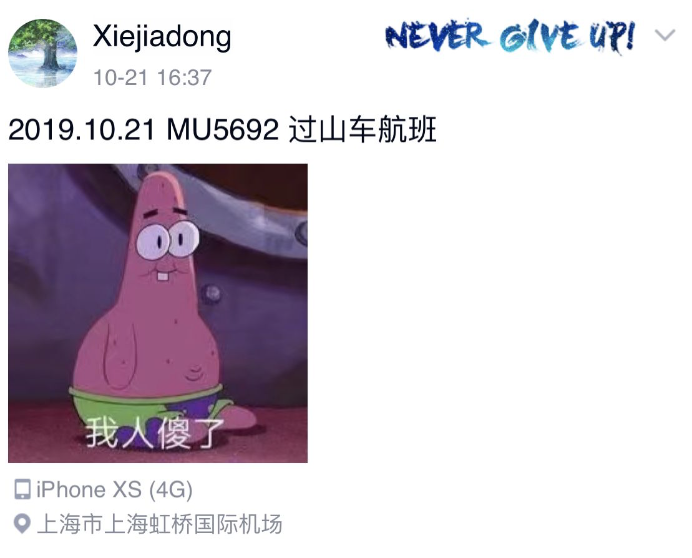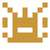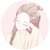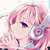# 2019.11 月赛题解

EOJ Monthly 招募贴

#### 花絮

• 题目背景是真的# Tag Idea Developer Tester
C 组合数学 Grunt Grunt 10185101232 Xiejiadong
E 筛法 Weaver_zhu Weaver_zhu 10185101232

### Problem B

##### First Solved：emengdeath

• $|s|$ 表示字符集大小，当 $k\ge |s|-1$ 的时候，肯定是所有的字符串都能等价了。

oxx1108 ： 感觉这个得和出题人心灵相通才能做吧。

### Problem C

##### First Solved：DeaphetS

$[2n]$ 分为 $n$ 对，分别是 $(1,2),(3,4),\cdots,(2n-1,2n)$，易知对于任意对 $(2k-1,2k)$，有三种可能的情况：

1. 奇对。选 $2k-1$，不选 $2k$
2. 偶对。选 $2k$，不选 $2k-1$
3. 空对。$2k$$2k-1$ 都不选。

Double counting，从两个方向数有序对 $(A,E)$ 的数量，其中 $A$ 是一种子集取法，遍历 $g(n,r,s)$ 的所有解，$E$$A$ 中空对的编号，共有 $n-s-r$ 个可能的取值，考虑先枚举 $A$ 还是先枚举 $E$，可得

Grunt ：本题出自笔者修的一门组合数学课的课后作业，感觉这个问题和做法都很有意思，就稍微加强了下，搬运过来。

### Problem D

##### First Solved：ldxxx

• 特殊点与特殊点之间的边，需要计算边权；
• 特殊点与非特殊点之间的边，需要计算边权；
• 非特殊点与非特殊点之间的边，不需要计算边权。

### Problem E

##### First Solved：poorwill

Weaver_zhu ：很没意思的一道题，和多校的一道题套路也重的比较多。

### 恭喜 DeaphetS ， emengdeath ， interestingLSY 分别获得冠亚季军。• 总共有几个等价类
• 每个等价类中都有啥

• 新开一个等价类
• 将这个字母加入到之前的某一个等价类中